# How to Count Number of Cells that Contain Errors in Excel

This post will guide you how to count the number of cells that contain errors within a range of cells using a formula in Excel 2013/2016.You can easily to count cells equal to a specific value or text string through COUNTIF function. But if there is an easy way to count cells contain errors in your data range.

## Count Number of Cells that Contain Errors

You should know that there are several types of errors such as: `#N/A`, `#REF!` or `#VALUE!` In Excel. And you only want to count the number of all types of errors with a formula in Excel. This tutorial will show you how to count the number of cells in a specified range of cell A1:B6 that contain errors using an Excel formula ,with the SUMRODUCT and ISERROR functions. Like below:

=SUMPRODUCT(–ISERROR(A1:B6))

###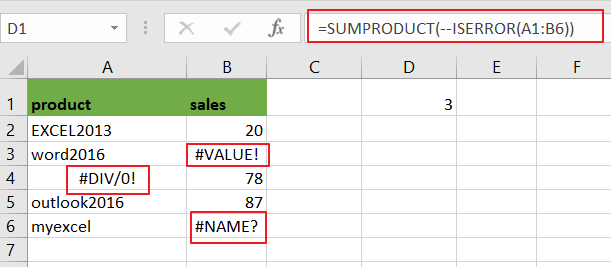Note: A1:B6 is the range from which to count the cells that contain errors.

LET’S SEE THAT HOW THIS FORMULA WORKS:

The SUMPRODUCT function can be used to multiply the corresponding components in the given arrays. If there is only one array, SUMPRODUCT function would return the sum of values in the array.

=ISERROR(A1:B6)

###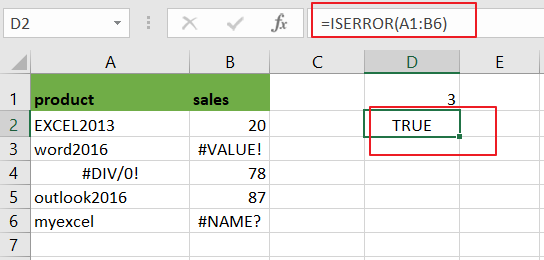The ISERROR function is used to identify the cells that contain an error. And returns True if cell contain any error values. In this case, ISERROR function returns an array that contain TRUE or FALSE values, like this:

={FALSE,FALSE;FALSE,FALSE;FALSE,TRUE;TRUE,FALSE;FALSE,FALSE;FALSE,TRUE}

=–ISERROR(A1:B6)

###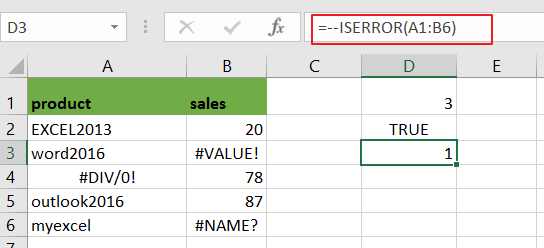You still need to convert the TRUE or FALSE values to number 1 and 0 by using a double negative operator. And the result array looks like this:

{0,0;0,0;0,1;1,0;0,0;0,1}

###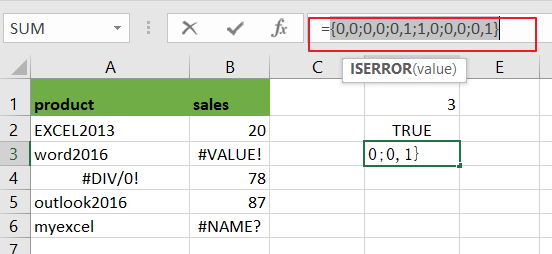The SUMPRODUCT function would add the value of the above array and return the final result.

You can also use another array formula based on the SUM function, IF function and ISERROR function to count the number of all types of errors in a range in Excel. Like this:

{=SUM(IF(ISERROR(A1:B6),1))}

###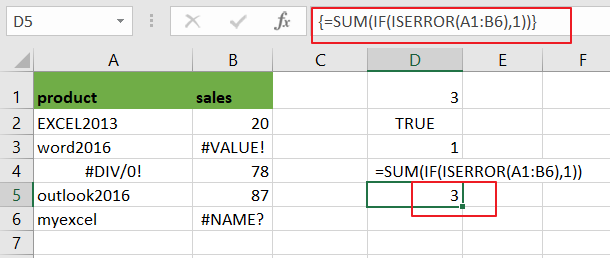Note: you need to press CTRL + SHIFT + ENTER short keys to covert its as a array formula.

### Related Functions

• Excel SUMPRODUCT function
The Excel SUMPRODUCT function multiplies corresponding components in the given one or more arrays or ranges, and returns the sum of those products. The syntax of the SUMPRODUCT function is as below:= SUMPRODUCT (array1,[array2],…)…
• Excel COUNTIF function
The Excel COUNTIF function will count the number of cells in a range that meet a given criteria. This function can be used to count the different kinds of cells with number, date, text values, blank, non-blanks, or containing specific characters.etc.= COUNTIF (range, criteria)…
• Excel IF function
The Excel IF function perform a logical test to return one value if the condition is TRUE and return another value if the condition is FALSE. The IF function is a build-in function in Microsoft Excel and it is categorized as a Logical Function.The syntax of the IF function is as below:= IF (condition, [true_value], [false_value])….
• Excel ISERROR function
The Excel ISERROR function used to check for any error type that excel generates and it returns TRUE for any error type, and the ISERR function also can be checked for error values except #N/A error, it returns TRUE while the error is #N/A. The syntax of the ISERROR function is as below:= ISERROR (value)….
• Excel SUM function
The Excel SUM function will adds all numbers in a range of cells and returns the sum of these values. You can add individual values, cell references or ranges in excel.The syntax of the SUM function is as below:= SUM(number1,[number2],…)…